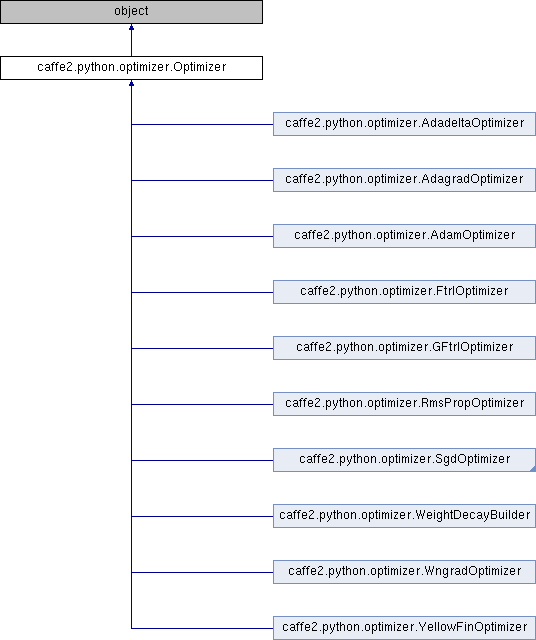Caffe2 - Python API A deep learning, cross platform ML framework
caffe2.python.optimizer.Optimizer Class Reference
Inheritance diagram for caffe2.python.optimizer.Optimizer:## Public Member Functions

def __init__ (self)

def __call__ (self, net, param_init_net, param, grad=None)

def get_cpu_blob_name (self, base_str, node_name='')

def get_gpu_blob_name (self, base_str, gpu_id, node_name)

def make_unique_blob_name (self, base_str)

def build_lr (self, net, param_init_net, base_learning_rate, learning_rate_blob=None, policy="fixed", iter_val=0, kwargs)

def get_auxiliary_parameters (self)

def scale_learning_rate (self, args, kwargs)

def create_lars_inputs (self, param_init_net, weight_decay, trust, lr_max)

## Detailed Description

Definition at line 30 of file optimizer.py.

## Member Function Documentation

 def caffe2.python.optimizer.Optimizer.add_lr_multiplier ( self, lr_multiplier )
```Set the global learning rate multiplier. If a multiplier already
existed, this will overwrite the existing multiplier. The multiplier is
used for all future calls to _run(), unless it is overwritten.
```

Definition at line 147 of file optimizer.py.

 def caffe2.python.optimizer.Optimizer.get_auxiliary_parameters ( self )
```Returns a list of auxiliary parameters.

Returns:
aux_params: A namedtuple, AuxParams.

aux_params.local stores a list of blobs. Each blob is a local
auxiliary parameter. A local auxiliary parameter is a parameter in
the local auxiliary parameter is the squared sum parameter, because
every learning rate has a squared sum associated with it.

aux_params.shared also stores a list of blobs. Each blob is a shared
auxiliary parameter. A shared auxiliary parameter is a parameter
that is shared across all the learning rate parameters. Take adam as
an example, the iteration parameter is a shared parameter, because
all the learning rates share the same iteration parameter.
```

Definition at line 181 of file optimizer.py.

 def caffe2.python.optimizer.Optimizer.make_unique_blob_name ( self, base_str )
```Returns a blob name that will be unique to the current device
and optimizer instance.
```

Definition at line 75 of file optimizer.py.

The documentation for this class was generated from the following file: